Teach
With
Bigshot
 Prev Introduction      Pinhole      Refraction      Lens Basics      Thin Lens Lens Properties      Focusing      Image Appearance      Illustrations Next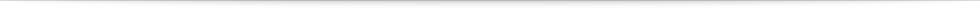### Illustrations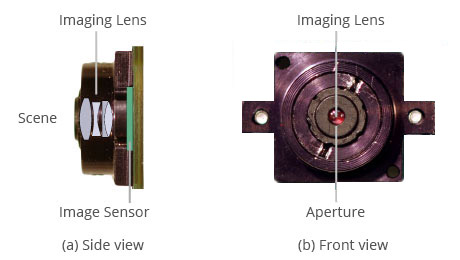Figure 1: Bigshot's lens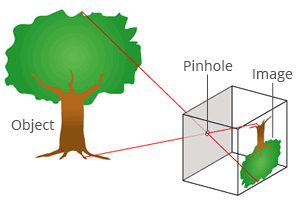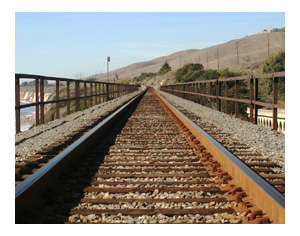Figure 2: Pinhole camera Figure 3: Perspective distortion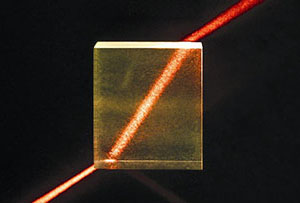Figure 4: Refraction through a glass slab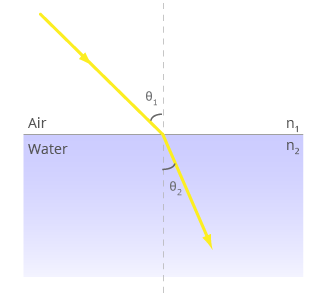Figure 5: Geometry of refraction
 θ1 θ2 n1 n2 Angle of incidence (θ1=45º) Angle of refraction (θ2=45º) Air (n1 = 1) Water (n1 = 1.33) Glass (n1 = 1.52) Sapphire (n1 = 1.77) Diamond (n1 = 2.4) Air (n2 = 1) Water (n2 = 1.33) Glass (n2 = 1.52) Sapphire (n2 = 1.77) Diamond (n2 = 2.4) Figure 6: Select a material to see how it bends light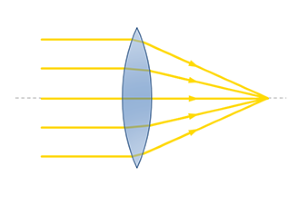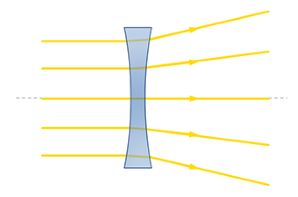Figure 7a: Convex lens converges light Figure 7b: Concave lens diverges light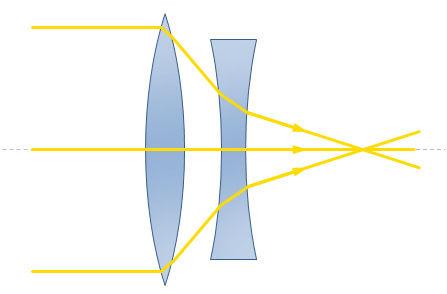Figure 8: A compound lens comprised of a convex and a concave lens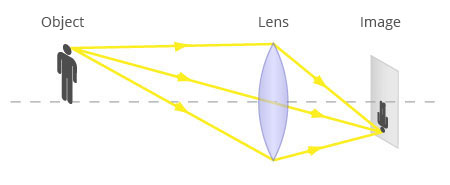Figure 9: Imaging using a thin convex lens
 Figure 10: Changing aperture of a lens using an iris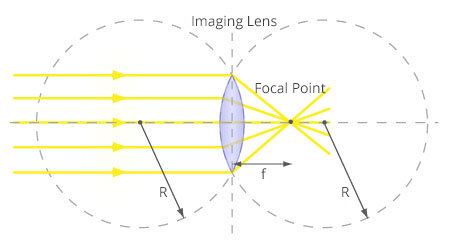Figure 11: Focal point of a convex lens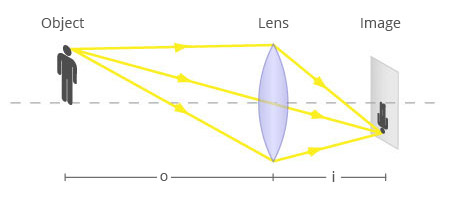Figure 12: Thin lens lawCar Position Sensor Position Aperture Size Figure 13: Image formation
 Prev Next